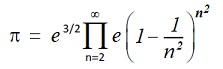# HP Forums

Full Version: Accurate x - log(1+x)
You're currently viewing a stripped down version of our content. View the full version with proper formatting.
(04-27-2020 07:54 PM)Albert Chan Wrote: [ -> ]I tried to re-use my formula, for log(probability of no repetition)

ln_P(n,s) := -s/(12*n*(n-s)) - (s + (n-s+0.5)*ln(1-s/n))

30 people birthday "collision" ≈ -expm1(ln_P(365,30)) = 0.706316242724
Using exact formula: ﻿ ﻿ ﻿ ﻿ ﻿ ﻿ ﻿ ﻿ ﻿ ﻿ ﻿1 - perm(365,30)/365^30 = 0.706316242719

For big n, small x = s/n, we can drop the first term.
Second term has a problem, even if we have accurate log1p

(s + n*ln(1 - s/n)) / n = x + log(1-x) ≈ x - x = 0

There might be a better way. For now, I use:

x + log(1-x) = x - (x + x^2/2 + x^3/3 + x^4/4 + ...) = -(x^2/2 + x^3/3 + x^4/4 + ...)

We have correct formula for ln_P(n,s), but numerically very hard to evaluate with big n.
It would be nice if we can accurately calculate x - log1p(x)

For small x, we can sum taylor/pade series. log1p(x) = 2*atanh(x/(x+2))

Code:
function tinyx_sub_log1p(x) --   x - log1p(x)     local y = x/(x+2)       -- = x - 2*atanh(y)     local z = y*y           -- = y*(x+2) - 2*(y + y^3/3 + y^5/5 + ...)     z = z*(1/3 + z*(1/5 + z*(1/7 + z/9 / (1-9/11*z))))     return y * (x-2*z)      -- relerr O(x^11/805376) end

y = x/(x+2) ≈ x/(0+2) = x/2
abs_err(z) ≈ (1/13 - 9/121) * y^12 ≈ (4/1573) * (x/2)^12 = x^12/1610752
(x-2z) ≈ x - 2/3*y² ≈ x - 2/3*(x²/4) = x - x²/6 ≈ x

relerr = abs_err(2z)/(x-2z) ≈ x^11/805376

relerr(x=0.1000) ≈ 1.24e-17, below Free42 Binary machine epsilon
relerr(x=0.0025) ≈ 2.96e-35, below Free42 Decimal machine epsilon

For big x, we use "half-angle" formula for log1p
Keep reducing log1p argument, until we can use tinyx_sub_log1p

log1p(x) = 2 * log1p(√(1+x) - 1) = 2 * log1p(x / (√(1+x) + 1))

Let y=sqrt(1+x), z = (y-1) = x/(y+1)
Let f(x) = x - log1p(x)

f(x) = z*(y+1) - 2*log1p(z) = z*(y-1) + 2*f(z) = z^2 + 2*f(z)

Both RHS terms are non-negative, without cancellation errors.
Code:
function x_sub_log1p(x)     if abs(x) < 0.1 then return tinyx_sub_log1p(x) end     x = x / (sqrt(1+x)+1)   -- "half-angle" formula     return x_sub_log1p(x)*2 + x*x end
(04-27-2020 07:54 PM)Albert Chan Wrote: [ -> ]I tried to re-use my formula, for log(probability of no repetition)

ln_P(n,s) := -s/(12*n*(n-s)) - (s + (n-s+0.5)*ln(1-s/n))

30 people birthday "collision" ≈ -expm1(ln_P(365,30)) = 0.706316242724
Using exact formula: ﻿ ﻿ ﻿ ﻿ ﻿ ﻿ ﻿ ﻿ ﻿ ﻿ ﻿1 - perm(365,30)/365^30 = 0.706316242719

Let's try our new x_sub_log1p function. (note: y = x - log1p(x) ≥ 0)
Code:
function ln_nr(n,s)         -- log(probability of no repetition)     local x = -s/n     local y = x_sub_log1p(x)     return x/(12*(n-s)) + n*y + (s-0.5)*(x-y) end function P_collision(n,s) return -expm1(ln_nr(n,s)) end

lua> P_collision(365, 30)
0.7063162427241915
lua> P_collision(2^128, 5e12)
3.6734198463188465e-014
lua> P_collision(2^128, 2.6153e18)
0.009999839905579181

Let's see what happen if we replace with naive implementation ...

lua> function x_sub_log1p(x) return x - log1p(x) end
lua> P_collision(365, 30) -- still OK, n is not huge
0.7063162427241918
7.346839692638293e-014
0.019899683013021148
From [VA] SRC #010 - Pi Day 2022 Special

(03-14-2022 08:03 PM)Valentin Albillo Wrote: [ -> ]unexpected infinite product for $$\pi$$ announced in the title, the awesome expression:which beautifully relates $$\pi$$ and e.
(03-22-2022 01:01 AM)Albert Chan Wrote: [ -> ]
(03-22-2022 12:14 AM)Valentin Albillo Wrote: [ -> ]To settle down the question, if someone with access to Mathematica or some other arbitrary-precision software can compute the product for N=100,000 using 100 digits, say, or as many as necessary to ensure full 34 correct digits or more, and post here the resulting value I'd appreciate it. Thanks in advance.

>>> from mpmath import *
>>> mp.dps = 100
>>> pn = lambda n: exp(nsum(lambda x: 1+log1p(-1/(x*x))*x*x,[2,n]) + 1.5)
>>> n = mpf(100000)
>>> N = 2*n+1
>>> x = pn(n)
>>> print x
3.141608361513791562872866895754895278060325823725833279147116393910631517290786764227775828378244404

lua> n = 100000
lua> s = 0
lua> for x=n,2,-1 do y=x*x; s = s + (1 + log1p(-1/y)*y) end
lua> exp(s + 1.5) -- error = -440 ULP
3.141608361513987

lua> s = 0
lua> for x=n,2,-1 do y=x*x; s = s - x_sub_log1p(-1/y)*y end
lua> exp(s + 1.5) -- error = 0 ULP
3.1416083615137915

Using x_sub_log1p(x), we get true PN, without ULP error !
We can use x_sub_log1p(), to get accurate (e^x - 1 - x), for small x

y = x - log(1+x)
e^y = e^x / (1+x)
(e^y - 1) = (e^x - 1 - x) / (1+x)

(e^x - 1 - x) = (e^y - 1) * (1+x)

Code:
function expm1_sub_x(x)     local z = expm1(x_sub_log1p(x))     return z + z*x end

lua> x = 1/1024
lua> expm1(x) - x      -- error = 967 ULP
4.769924165351404e-007
lua> expm1_sub_x(x) -- error = -1 ULP
4.769924165352429e-007

Comment: next post expm1_sub(x) take care of big x too.
It is also more consistent throughout, func_sub(x) = func(x) - x
Let f(x) = x - log(1+x)

f(x) - f(-x) = (x - log(1+x) - (-x - log(1-x)) = 2*(x - atanh(x))

LHS f() is never negative, thus possible massive cancellations.
We can go from accurate (atanh(x)-x) to accurate (log(1+x)-x), but not in reverse.
Code for RHS should be factored out.

Note that I flipped the order, to be consistent throughout, func_sub(x) = func(x) - x
I also use better pade approximation for atanh_sub_tiny(x), making it more useful by itself.

Code:
function atanh_sub_tiny(x)  -- abserr ≈ 256/2760615 * x^15     local y = x*x           -- pade(atanh(x)-x,x,14,8)     return x*y * (5005 - y*(5082 - y*969))                / (15015 - y*(24255 - y*(11025 - y*1225))) end function log1p_sub_tiny(x)  -- abserr ≈ -1/176679360 * (x/(1+x/2))^15     local y = x/(x+2)     return atanh_sub_tiny(y)*2 - x*y    -- approx -x*y ≤ 0 end function log1p_sub(x)       -- Free42 Decimal, 0.2 -> 0.01     if abs(x) < 0.2 then return log1p_sub_tiny(x) end     if x < -.79681 or x > 2.51286 then return log1p(x)-x end     x = x / (sqrt(1+x)+1)   -- "half-angle" formula     return log1p_sub(x)*2 - x*x end function expm1_sub(x)     if x > 1.25643 then return expm1(x) - x end     if x < -.76804 then return exp(x)-(1+x) end     local z = expm1(-log1p_sub(x))     return z + z*x end function ln_nr(n,s)         -- log(probability of no repetition)     local x = -s/n     local y = log1p_sub(x)     return x/(12*(n-s)) - n*y + (s-0.5)*(x+y) end

Example, expression ((1+r)^-n - 1)/r + n

lua> r, n = 1e-6, 1e3
lua> expm1(-n*log1p(r))/r + n --> error = 788 ULP
0.5003328749086222
lua> z = log1p_sub(r)
lua> (expm1_sub(-n*(z+r)) - n*z)/r --> error = -1 ULP
0.5003328749087098

lua> mapm = require'mapm'
lua> r = mapm.new(r) -- exact binary to decimal conversion
lua> (((1+r)^-n - 1)/r + n):tofixed(20)
0.50033287490870967825
I added HP71B implementation of log1p_sub(x), called FNL(X)